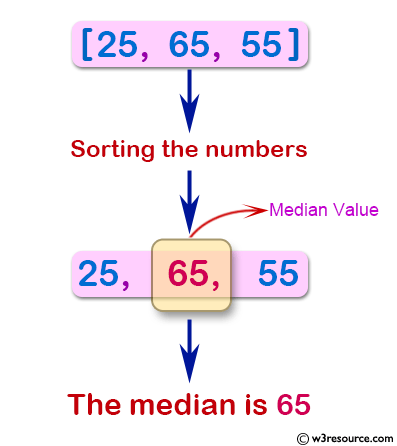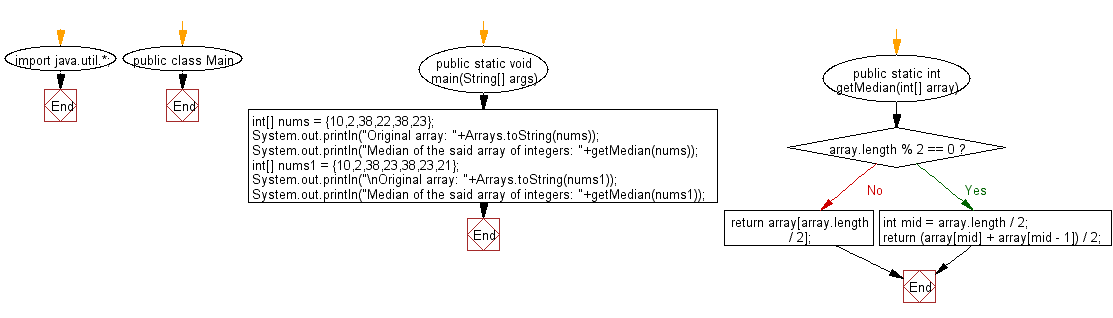﻿ Java: Median of a given unsorted array of integers# Java Exercises: Calculate the median of a given unsorted array of integers

## Java Basic: Exercise-128 with Solution

Write a Java program to calculate the median of a given unsorted array of integers.
Example: {10,2,38,23,38,23,21}
Output: 23

Pictorial Presentation:Sample Solution:

Java Code:

``````import java.util.*;
public class Main {
public static void main(String[] args)
{
int[] nums = {10,2,38,22,38,23};
System.out.println("Original array: "+Arrays.toString(nums));
System.out.println("Median of the said array of integers: "+getMedian(nums));
int[] nums1 = {10,2,38,23,38,23,21};
System.out.println("\nOriginal array: "+Arrays.toString(nums1));
System.out.println("Median of the said array of integers: "+getMedian(nums1));
}
public static int getMedian(int[] array) {
if(array.length % 2 == 0) {
int mid = array.length / 2;
return (array[mid] + array[mid - 1]) / 2;
}
return array[array.length / 2];
}
}
```
```

Sample Output:

```Original array: [10, 2, 38, 22, 38, 23]
Median of the said array of integers: 30

Original array: [10, 2, 38, 23, 38, 23, 21]
Median of the said array of integers: 23
```

Flowchart:Java Code Editor:

What is the difficulty level of this exercise?

Test your Programming skills with w3resource's quiz.

﻿

## Java: Tips of the Day

countOccurrences

Counts the occurrences of a value in an array.

Use Arrays.stream().filter().count() to count total number of values that equals the specified value.

```public static long countOccurrences(int[] numbers, int value) {
return Arrays.stream(numbers)
.filter(number -> number == value)
.count();
}
```

Ref: https://bit.ly/3kCAgLb